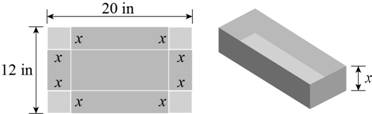# The function that models the volume of the box.### Precalculus: Mathematics for Calcu...

6th Edition
Stewart + 5 others
Publisher: Cengage Learning
ISBN: 9780840068071### Precalculus: Mathematics for Calcu...

6th Edition
Stewart + 5 others
Publisher: Cengage Learning
ISBN: 9780840068071

#### Solutions

Chapter 2, Problem 26P

(a)

To determine

## To find: The function that models the volume of the box.

Expert Solution

The function that models the volume of the box is V(x)=x(122x)(202x) .

### Explanation of Solution

Given:

The cardboard of the length 12in3 and breadth of 20in3 from which the four square has cut shown in the figure below.Figure (1)

Formula used:

The volume of the cuboids is,

V=l×b×h (1)

Calculation:

The length of the cardboard is 20in3 and has cut down the two square from the one length then the rest length of the box is,

lengthofthebox=(202x)in

The breadth of the cardboard is 12in3 and has cut down the two square from the one length then the rest length of the box is,

lengthofthebox=(122x)in

The height of the box is x in.

Substitute (202x) for l, (122x) for b and x for h in equation (1),

The volume of the cuboids is,

V=(202x)(122x)x

Thus, function that models the volume of the box is V(x)=x(122x)(202x) .

(b)

To determine

### To find: The values of x for which the volume is greater than 200 in3 .

Expert Solution

The values of x should be greater than 50in and 22.28in .

### Explanation of Solution

From the part (a), the function that models the volume of the box is V(x)=x(122x)(202x) .

Substitute 200 for V(x) in the volume V(x)=x(122x)(202x) and greater than to the R.H.S.

x(122x)(202x)>2004x(x216x+60)>200

Further solve the above inequality,

4x>200x>50

And,

x216x+60>200x216x140>0

Now use the quadratic formula to solve the above equation,

x=b±b24ac2a (2)

Compare the quadratic equation (1) with the general form of the quadratic equation ax2+bx+c=0 , then

a=1,b=16,c=140

Substitute 16 for b, 1 for a and 140 for c and x2 for x in equation (2),

x=(16)±(16)2+4114021=16±256+5602=16±8162

Take positive and negative of the above equation,

x=16+8162=22.28

And,

x=168162=6.28

Since the negative length is possible.

Thus, the value of x is 50in and 22.28in .

(c)

To determine

### To find: The largest volume of the box.

Expert Solution

The largest volume of the box is 352000in3 .

### Explanation of Solution

From the part (a), the function that models the volume of the box is V(x)=x(122x)(202x) .

Substitute 50 for x in the volume V(x)=x(122x)(202x)

V(50)=50(12250)(20250)=50(12100)(20100)=508880=352000

Substitute 22.28 for x in the volume V(x)=x(122x)(202x)

V(22.28)=22.28(12222.28)(20222.28)=22.2824.5632.56=17816.727

Thus, the largest volume is 352000in3 .

### Have a homework question?

Subscribe to bartleby learn! Ask subject matter experts 30 homework questions each month. Plus, you’ll have access to millions of step-by-step textbook answers!